# Discharging a Capacitor (Formula And Graphs)

## What is Discharging a Capacitor?

Discharging a capacitor means releasing the charge stored within the capacitor. Let’s go over an example where a capacitor is discharged.

We connect a charged capacitor of capacitance C farad in series with a resistor of resistance R ohms.

We then short circuit this series combination by switching on the push switch as shown.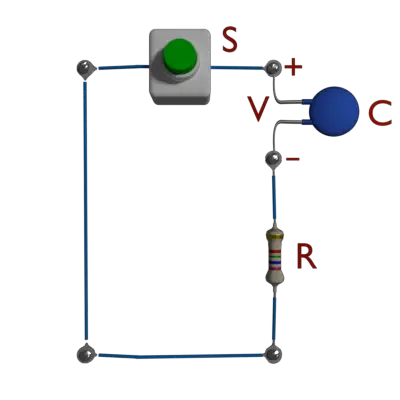As soon as the capacitor is short-circuited, it starts discharging.

Let us assume, the voltage of the capacitor at fully charged condition is V volt. As soon as the capacitor is short-circuited, the discharging current of the circuit would be – V / R ampere.

But after the instant of switching on that is at t = +0, the current through the circuit is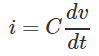As per Kirchhoff’s Voltage Law, we get,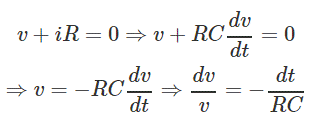Integrating both sides, we get,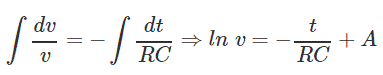Where, A is the constant of integration and, at t = 0, v = V,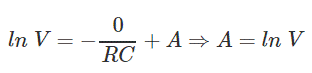After calculating the value of A, we get,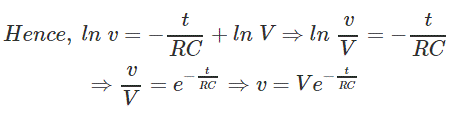We know the form, KVL of the circuit,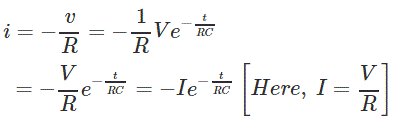If we plot these discharging current and voltage in the graph, we get,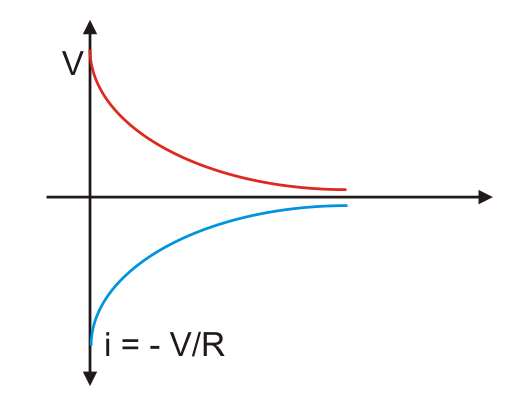Hence the capacitor current exponentially reaches zero from its initial value, and the capacitor voltage reaches exponentially to zero from its initial value during discharging.

Want To Learn Faster? 🎓
Get electrical articles delivered to your inbox every week.
No credit card required—it’s 100% free.